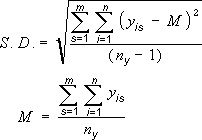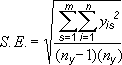# Equations for calculating the error amount

## Standard Deviationwhere:

s = series number

i = point number in series s

m = number of series for point y in chart

n = number of points in each series

yis = data value of series s and the ith point

ny = total number of data values in all series

M = arithmetic mean

## Standard Error